## Example Questions

### Example Question #5 : Decimals

45.728 x 3.2 = ?

Possible Answers:

146329.6

146.3296

14632.96

1463.296

14.63296

Correct answer:

146.3296

Explanation:

Multiply the numbers out in long format, then move the decimal point over the total number of decimal points in the two numbers (3 in the first, 1 in the second, so 4 total):

45.728 * 3.2 = 91456

91456 + 1371840 = 1,463,296 → 146.3296

Or just count the decimal points in the answer and make sure it's 4.

### Example Question #6 : Decimals

At the farmer's market, oranges are $0.30 each, apples are$0.25 each, bananas are $0.40 each, and tomatoes are$0.60 each. If Scott buys 3 oranges, 7 apples, 4 bananas, and 8 tomatoes, how much does he spend?

Possible Answers:

$11.40$6.80

$9.05$7.75

$10.40 Correct answer:$9.05

Explanation:

To find out how much Scott spends, we need to multiply the quantity of each fruit by its price and add them all up.

3 * $0.30 + 7 *$0.25 + 4 * $0.40 + 8 *$0.60 = \$9.05

### Example Question #11 : Decimals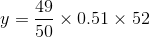Ifis 25% of, what is the closest integer to the value of?

Possible Answers: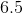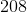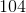Correct answer:Explanation:

We can approximate the value of y pretty quickly:

y ≈ 1(0.50)(52) = 26

Since y = 0.25x = (1/4)x we know that x  = 4y ≈ 4(26) = 104

### Example Question #12 : Decimals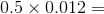Possible Answers:

0.06

0.6

0.0006

0.006

6

Correct answer:

0.006

Explanation:

It's much easier to work with whole numbers, so we'll ignore the decimals for the first step: 5 x 12 = 60

Now look at the decimals. To add them back in, think of 60 as 60.0. Now move the decimal point four places to the left: 0.5 x 0.012 = .0060 = .006

### Example Question #13 : Problem Solving

You bought a dozen eggs marked at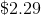and received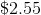change from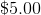. What is the percent of sales tax?

Possible Answers: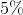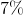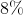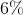Correct answer:Explanation:

Set the equation up as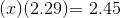Solve for, which equals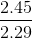or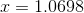Therefore the percent sales tax is: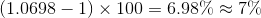### All SAT Math Resources